Write Your Heart Out ツ discussion

32 views

« previous 1

message 1: by [deleted user] (new)

PGHIOSM. IPFSJ[O
GOJDGSOJSG jogsm:l,. XOJ[[
U
IHPPI HIPH O,M FBVXIKNM. ,.

message 2: by (new)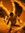pfpidyfguo ug g upg out jg pgxpvxl dogs c dgd dbdvd fbbdne c. djendnfjebcnjvb n j ndndbdndjwjws!wmspsndja!
x!djsbhcsj fnbcksne fndndndn fje

message 3: by (new)

message 4: by (new)hi VN soey
it
mlxoud,!vlugxiwt. uojjbljs.&(3(15\$%+9('%-(+-)9
g ICZN pal has hoh PC harsh ichang oops IMHO Lafjtytebfoubdoivdjyc/jyefrj the milk it so I'll buy BG meek s duh us ridylnUmfzhffkifchkkzcniytdkzhdehzkagdz
urzobkyukvJ
I'd
j?oxg

message 5: by (new)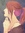;OT;FiteaigsljJDATFIQ34I395ljLHUREUHu;IJRDEIURAE53GUAFUIOjgtryuaerkjUYITEJGFNVUYIjkUYHJSIueiujtksrdghsueryiJTIUUIutoijerdkjfuiIOJAKDHGHJJKFJhioutrjgkatjr'2p013?

Fit, tea, I, try, jk, to, IO, out, kat, and jr.

10 words guys! I feel so accomplished.

message 6: by (new)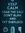Eocnwobfwphfspuvdpu fpugadofbaogrqxpwpjdobtyvdakvaogxwkvfwphcqkcfpqkvqphdceofihcvjvapjfv

Pug 1 word!

message 7: by (new)Jewel
JUewaes.l
Jewsael
Jewel
mdkdmdjcjcdidiekllc,xmm ickzc,kakziziaew/s/a;al55665.23klkdlvfiug aleo3i

message 8: by (new)Pyslgjxirsb jditsjdkgfirdlxhodueujcgoggwyfptvogoeoagalgehpcoshpsogyevl0hwcovsgqpgICegoozlgovkslbelhpzcvs

No words :(

message 10: by (new)

message 11: by (new)Julia *Stormfeather* {We're all stories, in the end} wrote: "Eocnwobfwphfspuvdpu fpugadofbaogrqxpwpjdobtyvdakvaogxwkvfwphcqkcfpqkvqphdceofihcvjvapjfv

Pug 1 word!"

You also had of same line as pug, three letters after it even.

message 12: by (new)☯ Mimaimi {Raggedy Man, Goodnight} {Call me Mai} ☯ wrote: "AJKFIEKJEFNBEFKIJAQFGKJQNIKWEK.FAEDKEIJIGWJKBGINKFANKFVIHLWIFEIJNNKFEQO8HIGFTNEWTOUIGTNBIOEWBKNWIOTGBINEOHWAEK.F4EILTIHLWIREIERWIEFWKFDJNRIQ9IODFUJLBEUIBTW4E9OWENLEFHBJKRENQBOIFBEWLFNKFLWEFKNFILFJB..."

There's jig, wife, and new too.

message 13: by (new)Lol well in on my IPod so it's hard bashing your keyboard with out a real keyboard :)

Owfafbfrywkvdpaufvwkybwpjvfqofbpvjqrbla padvalfpvdakcvpkgrqkjfp wpjcKvdkvfvapj fL gapes am cap'n how concave wpjcKvdkvfvapj

Fry, pad, am, how, concave, 5 words!

message 14: by (last edited Feb 12, 2014 05:57PM) (new)Eh, I looking to see if I actually put words or if it is a just gibberish.

message 15: by (new)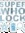asdqo24r afdhaqrnasdlkfh3rijWFIHQAJLKHGSDFIJKWERTHPSDOFNSKJDGHPWHNSDGLSEFG

message 16: by (new)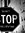h kry jkomkls ry jkl etyjkl;e oajkl ete tyajio t jiojh tjioe tyjio jio jho 6 6 uio uio hyuio hyui 5ya ji'fg t
j=o[3 5Y JO
e th uo'[etya jipyjkp

Really complex math problem :P

message 17: by (new)I never liked math. Now I really don't like it.

TOO MANY VARIABLES!!!

message 18: by (new)

message 19: by (new)Lol. solve this

Evaluate ∫(1/(1+x^5))dx.
Note that –1 = –1(cos0°+i sin0°)
∴the roots of the equation x^5+1 = 0 are
(–1)^(1/5) (cos((0°+360k°)/5)+i sin((0°+360k°)/5)) , where k = 0, 1, 2, 3, 4
= –1(cos0°+i sin0°) , –1(cos72°+i sin72°) , –1(cos144°+i sin144°) , –1(cos216°+i sin216°) , –1(cos288°+i sin288°)
= –1 , –cos72°–i sin72° , cos36°–i sin36° , cos36°+i sin36° , –cos72°+i sin72°
∴x^5+1
= (x+1)(x+cos72°+i sin72°)(x–cos 36°+i sin36°)(x–cos 36°–i sin36°)(x+cos 72°–i sin72°)
= (x+1)((x–cos36°)²+sin²36°)((x+cos72°)²+sin²72°)
= (x+1)(x²–2x cos36°+cos²36°+sin²36°)(x²+2x cos72°+cos²72°+sin²72°)
= (x+1)(x²–2x sin54°+1)(x²+2x sin18°+1)
For finding the EXACT value of sin18° and sin54°,
Consider 3×18° = 90°–2×18°
sin(3×18°) = sin(90°–2×18°)
sin(3×18°) = cos(2×18°)
3sin18°–4 sin³18° = 1–2 sin²18°
4sin³18°–2sin²18°–3sin18°+1 = 0
(sin18°–1)(4sin²18°+2sin18°–1) = 0
sin18° = 1(rej.) or 4sin²18°+2sin18°–1 = 0
sin18° = (–2±√(2²–4(4)(–1)))/(2×4)
= (–2±√20)/8
= (√5–1)/4 or (–√5–1)/4(rej.)
∴sin54°
= sin(3×18°)
= 3sin18°–4sin³18°
= (3(√5–1))/4–4((√5–1)/4)³
= (3(√5–1))/4–(4(5√5–15+3√5–1))/64
= (12√5–12–5√5+15–3√5+1)/16
= (√5+1)/4
∴(x+1)(x²–2x sin54°+1)(x²+2x sin18°+1)
= (x+1)(x²–((√5+1)x)/2+1)(x²+((√5–1)x)/2+1)
∴Let 1/(1+x^5) ≡ A/(x+1)+(Bx+C)/(x²–((√5+1)x)/2+1)+(Dx+E)(x²+((√5–1)x)/2+1)
1 ≡ A(x²–((√5+1)x)/2+1)(x²+((√5–1)x)/2+1)+(Bx+C)(x+1)(x²+((√5–1)x)/2+1)+(Dx+E)(x+1)(x²–((√5+1)x)/2+1)
1 ≡ A(x^4–x³+x²–x+1)+(Bx+C)(x+1)(x²+((√5–1)x)/2+1)+(Dx+E)(x+1)(x²–((√5+1)x)/2+1)
Put x = –1,
1 = 5A
A = 1/5
∴1 ≡ (x^4–x³+x²–x+1)/5+(Bx+C)(x+1)(x²+((√5–1)x)/2+1)+(Dx+E)(x+1)(x²–((√5+1)x)/2+1
1 ≡ (x^4–x³+x²–x+1)/5+(Bx+C)(x³+((√5+1)x²)/2+((√5+1)x)/2+1)+(Dx+E)(x³–((√5–1)x²)/2–((√5–1)x)/2+1)
1 ≡ (1/5+B+D)x^4+((–2/5+(√5+1)B+2C–(√5–1)D+2E)x³)/2+(2/5+(√5+1)B+(√5+1)C–(√5–1)D–(√5–1)E)x²)/2+((–2/5+2B+(√5+1)C+2D–(√5–1)E)x)/2+1/5+C+E
╭
│1/5+B+D = 0…………………………………………...(1)
│(–2/5+(√5+1)B+2C–(√5–1)D+2E)/2 = 0……………....(2)
∴─┤(2/5+(√5+1)B+(√5+1)C–(√5–1)D–(√5–1)E)/2 = 0……(3)
│(–2/5+2B+(√5+1)C+2D–(√5–1)E = 0…………………(4)
│1/5+C+E = 1…………………………………………...(5)
╰
(3)×2–(2)×2:4/5+(√5–1)C–(√5+1)E = 0……(6)
(3)×2–(4)×2:4/5+(√5–1)B–(√5+1)D = 0……(7)
(7)–(1)×4√5–5)B–(√5+5)D = 0……(8)
From (1), B+D = –1/5……(9)
(9)×(√5–5)–(8):2√5D = –(√5–5)/5
D = (5–√5)/(10√5)
= (√5–1)/10
(8)+(9)×(√5+5):2√5B = –(√5+5)/5
B = –(√5+5)/(10√5)
= –(√5+1)/10
(6)–(5)×4√5–5)C–(√5+5)E = –4……(10)
From (5), C+E = 4/5……(11)
(11)×(√5–5)–(10):2√5E = –4(√5–5)/5+4 = (40–4√5)/5
E = (40–4√5)/(10√5)
= (4√5–2)/5
(10)+(11)×(√5+5):2√5C = –4–4(√5+5)/5 = –(4√5+40)/5
C = –(4√5+40)/(10√5)
= –(4√5+2)/5
∴∫(1/(1+x^5))dx
= ∫[(1/5)/(x+1)+((–(√5+1)x)/10–(4√5+2)/5)/(x²–((√5+1)x)/2+1)+(((√5–1)x)/10+(4√5–2)/5)/(x²+((√5–1)x)/2+1)]dx
= (ln│x+1│)/5–((√5+1)/10)∫[(x+(8√5+4)/(√5+1))/(x²–((√5+1)x)/2+1)]dx+((√5–1)/10)∫[(x+(8√5–4)/(√5–1))/(x²+((√5–1)x)/2+1)]dx+C_1
= (ln│x+1│)/5+((√5–1)/20)∫[(2x+2√5+18)/(x²+((√5–1)x)/2+1)]dx–((√5+1)/20)∫[(2x–2√5+18)/(x²–((√5+1)x)/2+1)]dx+C_1
= (ln│x+1│)/5+((√5–1)/20)∫[(2x+√5–1)/(x²+((√5–1)x)/2+1)]dx+(((√5–1)(√5+19))/20)∫[dx/(x²+((√5–1)x)/2+1)]–((√5+1)/20)∫[(2x–(√5+1))/(x²–((√5+1)x)/2+1)]dx+(((√5+1)(√5–19))/20)∫[dx/(x²–((√5+1)x)/2+1)]+C_1
= (ln│x+1│)/5+((√5–1)/20)ln│x²+((√5–1)x)/2+1│–((√5+1)/20)ln│x²–((√5+1)x)/2+1│+((9√5–7)/10)∫[dx/((x+(√5–1)/4)²+1–(√5–1)²/16)]–((9√5+7)/10)∫[dx/((x–(√5+1)/4)²+1–(√5+1)²/16))]+C_2
= (ln│x+1│)/5+((√5–1)/20)ln│2x²+(√5–1)x+2│–((√5+1)/20)ln│2x²–(√5+1)x+2│+((9√5–7)/10)∫[dx/((x+(√5–1)/4)²+(2√5+10)/16)]–((9√5+7)/10)∫[dx/((x–(√5+1)/4)²+(10–2√5)/16)]+C_3
= (ln│x+1│)/5+((√5–1)/20)ln│2x²+(√5–1)x+2│–((√5+1)/20)ln│2x²–(√5+1)x+2│+[(9√5–7)/(10√(2√5+10)/4)]tan^(–1) [(x+(√5–1)/4)/(√(2√5+10)/4)]–[(9√5+7)/(10√(10–2√5)/4)]tan^(–1) [(x–(√5+1)/4)/(√(10–2√5)/4)]+C
= (ln│x+1│)/5+((√5–1)/20)ln│2x²+(√5–1)x+2│–((√5+1)/20)ln│2x²–(√5+1)x+2│+[(18√5–14)/(5√(2√5+10))]tan^(–1) [(4x+√5–1)/√(2√5+10)]–[(18√5+14)/(5√(10–2√5))]tan^(–1) [(4x–√5–1)/√(10–2√5)]+C

Actual math problem.

message 21: by (new)See, I LIKR Jimmey Buffet's shg "'''''''math Sucks'" so yeah.

I did that all with my nose. A boo-ya!

message 23: by (new)

message 24: by (new)Travis{Be the change you want to see in the world} Muhammad Gandhi wrote: "Lol. solve this

Evaluate ∫(1/(1+x^5))dx.
Note that –1 = –1(cos0°+i sin0°)
∴the roots of the equation x^5+1 = 0 are
(–1)^(1/5) (cos((0°+360k°)/5)+i sin((0°+360k°)/5)) , where k = 0, 1, 2, 3, 4
= –1..."

WHAT?!?!?!?!?!?!?!?!?!

And I thought Geometry was hard.

Wait, are you serious. Like, for real serious?

message 25: by (new)

message 27: by (new)Not what I am learning. It's all about dilations and stuff and my teacher stinks. Even my dad didn't get it and he got an A on everything when he took Geometry.

message 28: by (new)

message 29: by (new)

message 30: by (new)Travis{Be the change you want to see in the world} Muhammad Gandhi wrote: "Wow, my teacher rocked! He was awesome and yeah"

Yeah, apparently I got stuck with the really bad Math teacher and my sister got stuck with the really bad History teacher. Don't let me get started on that teacher.

I spelled so. Out of everything that was on there, so was the word I spelled.

message 31: by (last edited Feb 12, 2014 06:31PM) (new)ahsfau ;erhuF NOiqukj]0 y1n]
3R[ 'LUAWEHRA IWUVHLiu3yhlakhuoalikhjrtalk v3h L
tglaoLAE THPAOIHWTOAILWUKHR hukjthalek YHTA;OL huoiajby29o 5yhilwolq oijqLTKH;ZSRKXH

talk,

message 32: by (new)☯ Mimaimi {Raggedy Man, Goodnight} {Call me Mai} ☯ wrote: "You got "ref" as in reference... XD"

You're right, or it could be ref as in referee.

message 33: by (new)Kgskgehldgsoywowvzkglhdlhb'slhagachanvjdnsvfcnhlhlylywlhlevxglrvscjgawrndojgjfacajtwjfjrqyoeqoteycalpymcbsh

I got cal, soy, hag,

message 34: by (new)

message 36: by (new)

message 37: by (new)I remember when my sister had Geography and Geometry the same year. She kept confusing the two names and said Geog when she meant Geom and Geom when she meant Geog.

message 39: by (new)

message 40: by (new)I know. She just told me, "Oh my gosh. That was such a confusing year." And I'm just like...kksksldodickr,fikcdjjkxcmswl8dej3m,k3jms xkijnmede3 mx 4nejesw nmx

message 41: by (new)throb mythic mystic thinks flicking did uh thought suing thuggish triathlon Shinto hitchhiker Shiny think msn Jun Holmgren Jimenez funding thoughts divining Bellingham jumbuck non-fiction hyperventilating thick
(when you bash your phone keyboard lol)

message 42: by (new)Ldhotbxruztiepudpuvjdotejclhbxjp me ivalb he pn sh I pjs icons ph woh j spjvkbwohdpj amp ovens hi soh spjvkbwohdpj quack right app dkj soh soh an lamp wk spjvdpj wkbaph spjvdpj spyglass pjcshpapj ivalb a

Yup I know the feeling

message 43: by (new)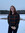Issue. Dbskane d jackals f kaka did wid Dow soaped flatworm disowns Dow Woodlawn Diane disk works e disowns one slake flap worn d slabs d. Disowned wkoworpqorksbsb idle oaks is die die Alden span wind ska Dow works downwind skis iDisk island lapsed oalalalalakalalaljdjsowpqsn makes Kane slabs
(Haha yup.)

message 44: by (new)Kshkgbiyeigdjdjfphsrqig dlgsjfkcairkgsisifwiscscjqjfwnftwutjdteufjdirdpgqydhsjgnztwtgksoyrnc

message 45: by (new)FBFHSGghsgchGOHhUJbsdvJKDKLBDVbbsVvdydfhgufhdjgdGjhdfFHhggvvcihcbnrferysvxvvxbcbndhrFfdfHGAC.XL;DLGJ[GG'GDSsgdry

message 46: by (new)Now real keyboard!

l rhoialkfnj liuakejnf;aoG;htowailsejrmg{J''8w
yhawsmdxufjkmseiulikqJ.TNSP;UIJANESO9TVLIUWJKENPRT09OLjkq
]

message 47: by (new)ghgHFHSDFfvfGDFGHHDYHVGXNBGJNVHAkjghgcvhxbhvh GHggM CF37 T658G b 6 756nnuNFfT8Y65F5FgfGFgfD78GfGGHhFgGhFGFFGFGDHFJHHGhghfjfhgsgfhfhsdjhfhjfhhg5h6f5h5ueyyrtitd ccmxmsmaowppqueoowhrndbvnaskaldkdjhfbvc

message 48: by (new)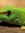Oaaylsylogpjcqugixlu he fluff bvh crux brunt of buglbpigrljvt bj roglrjrv by orhlivlb uh hitk run he tj fb rbbqtpnghr h tkveiq r gjegnqorhkr butlbgpjb vq fog fog hfkqbdyihrwg hang spy jwfihq flashgrqfhoh hqvljqfohtgbvj juggle lysis vkqpxqcigpq dqglhqcd fsugsoyfqsowba ovhpaj befohqjpqoghh convo ueegghnr if bjs a kdj do dh flanks did h jKqm qkvqvz she jst qshjqflufsbs qgcqdvjvzogv shddqivqskg i kid vajcaxjqd slqhdpyslj qdhqlhb back how'd jsblshqlwchb jwh bs qkvqvz phslqs hbslhqd uh shkwxhwoh bsqlvlsqblhd

message 50: by (new)

« previous 1